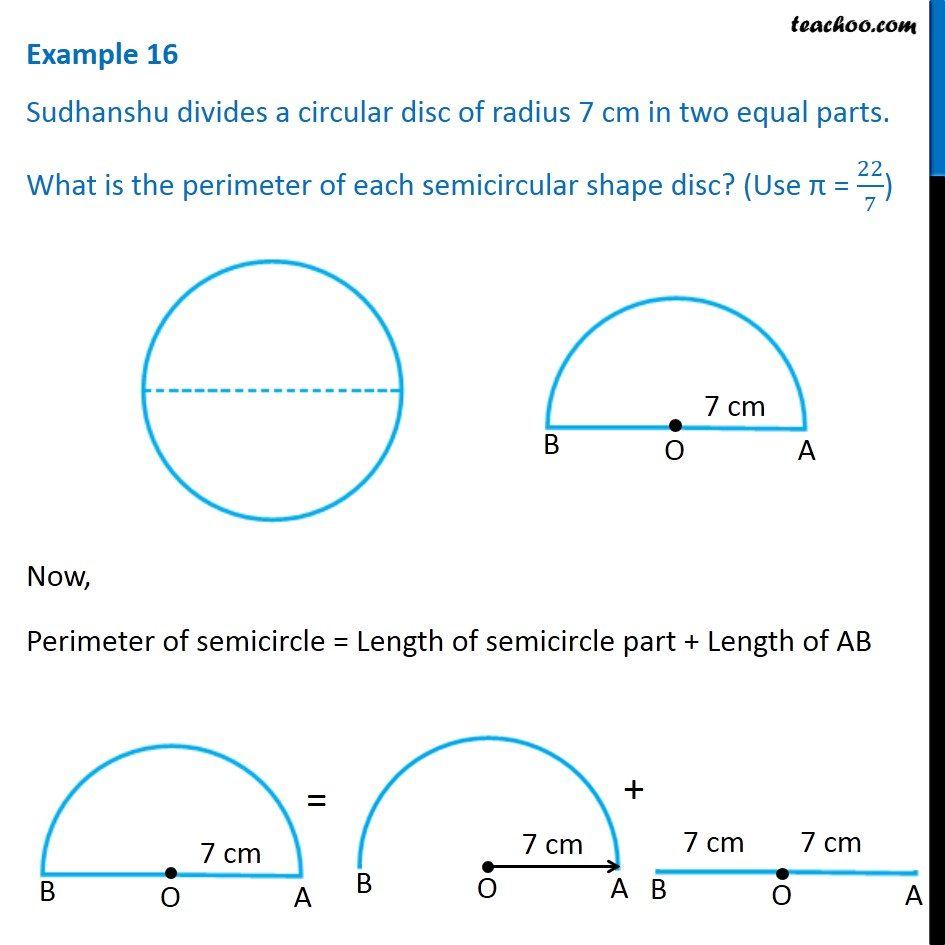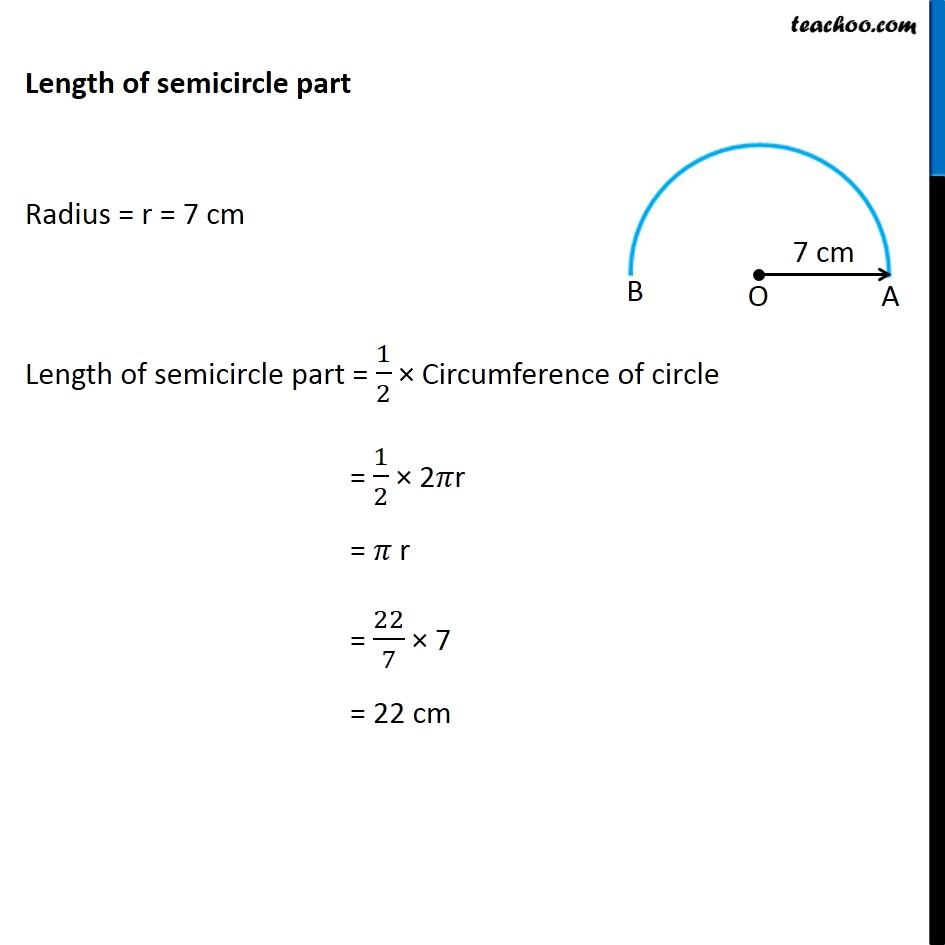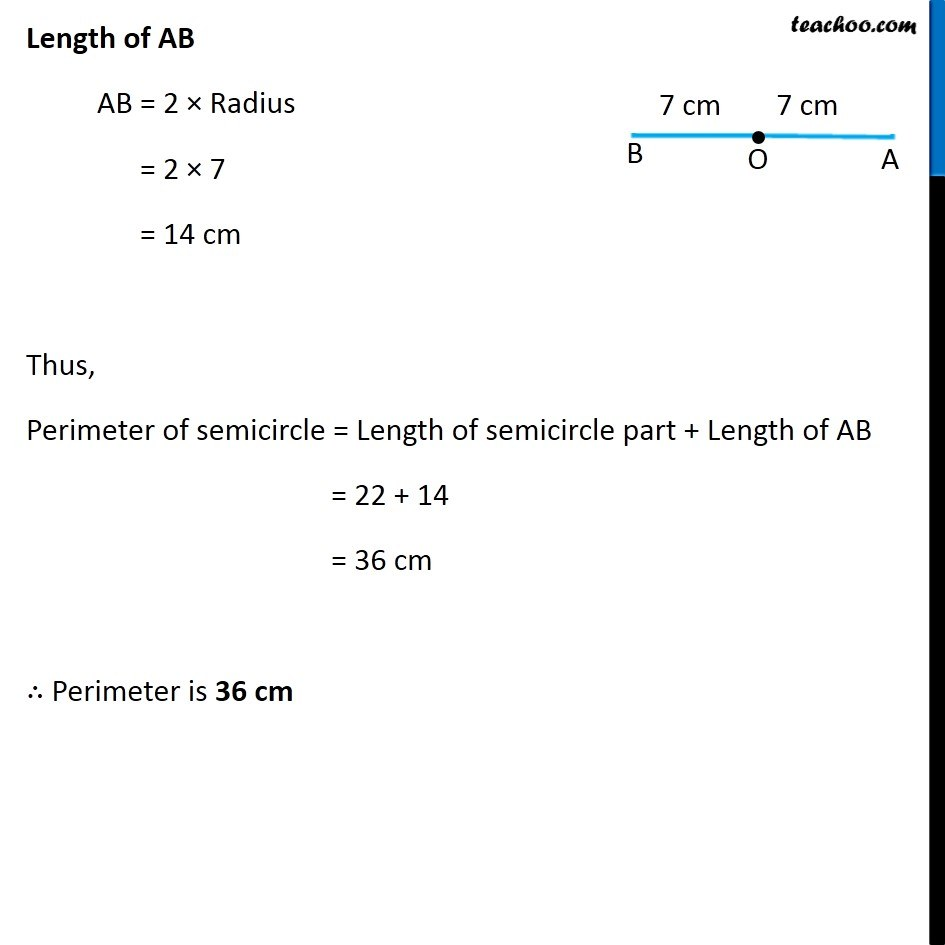1. Chapter 11 Class 7 Perimeter and Area
2. Concept wise
3. Perimeter of a combination of shapes

Transcript

Example 16 Sudhanshu divides a circular disc of radius 7 cm in two equal parts. What is the perimeter of each semicircular shape disc? (Use π = 22/7) Now, Perimeter of semicircle = Length of semicircle part + Length of AB Length of semicircle part Radius = r = 7 cm Length of semicircle part = 1/2 × Circumference of circle = 1/2 × 2𝜋r = 𝜋 r = 22/7 × 7 = 22 cm Length of AB AB = 2 × Radius = 2 × 7 = 14 cm Thus, Perimeter of semicircle = Length of semicircle part + Length of AB = 22 + 14 = 36 cm ∴ Perimeter is 36 cm

Perimeter of a combination of shapes